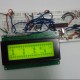February 09, 2010 by banerjenHi, I'm having a problem in using PC4 and PC5 as an I/P pin. I used the code -> ``````PORTC &= ~((1<> PC4; inp2 = (PINC & (1<> PC5; if (inp1) // print inp1 value on the LCD if (inp2) // print inp2 value on the LCD } `````` Now, the problem is that it is cycling between 1 and 0 for both the pins in the LCD irrespective of the input given to the PIN. Nandan. I'm assuming you're using a switch through a resistor to ground for your input or some other method of pulling the two pins you are checking low? If so, did you set your data direction registers (DDRC) and turn on the internal pullups? the code snippet below would do that for the two pins you want to check. ``````DDRC &= ~(1<